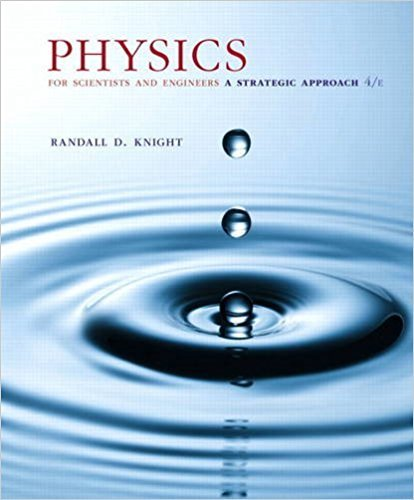×
×

# Blocks of mass m1 and m2 are connected by a masslessISBN: 9780134081496 191

## Solution for problem 12.112 Chapter 12

Physics for Scientists and Engineers: A Strategic Approach, Standard Edition (Chs 1-36) | 4th Edition

• Textbook Solutions
• 2901 Step-by-step solutions solved by professors and subject experts
• Get 24/7 help from StudySoup virtual teaching assistantsPhysics for Scientists and Engineers: A Strategic Approach, Standard Edition (Chs 1-36) | 4th Edition

4 5 0 431 Reviews
30
3
Problem 12.112

Blocks of mass m1 and m2 are connected by a massless string that passes over the pulley in Figure P12.65. The pulley turns on frictionless bearings. Mass m1 slides on a horizontal, frictionless surface. Mass m2 is released while the blocks are at rest. a. Assume the pulley is massless. Find the acceleration of m1 and the tension in the string. This is a Chapter 7 review problem. b. Suppose the pulley has mass mp and radius R. Find the acceleration of m1 and the tensions in the upper and lower portions of the string. Verify that your answers agree with part a if you set mp = 0.

Step-by-Step Solution:
Step 1 of 3
Step 2 of 3

Step 3 of 3

##### ISBN: 9780134081496

The full step-by-step solution to problem: 12.112 from chapter: 12 was answered by , our top Physics solution expert on 12/28/17, 08:06PM. This textbook survival guide was created for the textbook: Physics for Scientists and Engineers: A Strategic Approach, Standard Edition (Chs 1-36), edition: 4. Since the solution to 12.112 from 12 chapter was answered, more than 415 students have viewed the full step-by-step answer. Physics for Scientists and Engineers: A Strategic Approach, Standard Edition (Chs 1-36) was written by and is associated to the ISBN: 9780134081496. The answer to “Blocks of mass m1 and m2 are connected by a massless string that passes over the pulley in Figure P12.65. The pulley turns on frictionless bearings. Mass m1 slides on a horizontal, frictionless surface. Mass m2 is released while the blocks are at rest. a. Assume the pulley is massless. Find the acceleration of m1 and the tension in the string. This is a Chapter 7 review problem. b. Suppose the pulley has mass mp and radius R. Find the acceleration of m1 and the tensions in the upper and lower portions of the string. Verify that your answers agree with part a if you set mp = 0.” is broken down into a number of easy to follow steps, and 109 words. This full solution covers the following key subjects: . This expansive textbook survival guide covers 42 chapters, and 4463 solutions.

Unlock Textbook Solution